==提示：为方便阅读，《deeplearning.ai深度学习笔记》系列博文已统一整理到 http://dl-notes.imshuai.com==

Basics of Neural Network Programming

# Logistic Regression as a Neural Network

## Binary Classification

1. Example: 给一张64x64像素的图片图片，判断是否含有猫
2. 获取图片的RGB像素值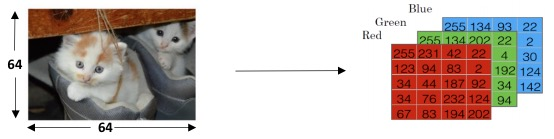3. 并unroll成一个vector $$X^{(i)}$$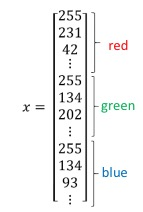4. 所有的vector组成数据集矩阵$$X$$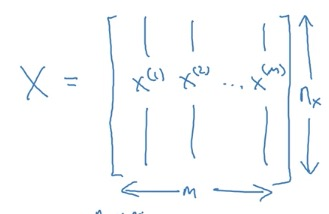特别注意，$$X$$的行是$$n$$，列是$$m$$，和Machine learning中的定义正好是转置的关系。这样有个好处，每条测试集在矩阵中都是以列向量的形式存在。

5. DeepLearning常用Notations:
• $$m$$: number of examples in datasets
• $$n_x$$: input size（即feature的个数）
• $$n_y$$: output size（即分类个数）
• $$X \in \mathbb{R}^{n_x\times m}$$ ：the input matrix
• $$Y \in \mathbb{R}^{n_y\times m}$$ ：the input matrix
• 带括号的上标$$^{(i)}$$，表示和training example相关的计数

1. 使用Python中的reshape方法，整理矩阵的维度。

## Logistic Regression

1. 问题描述： Logsitic Regression要求输出y不是0就是1。The goal of logistic regression is to minimize the error between its predictions and training data.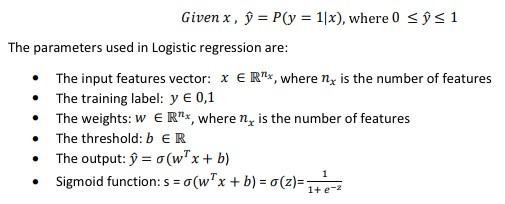2. sigmoid function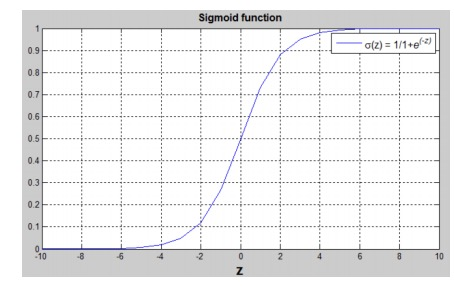==这里有个疑问，为什么sigmoid处理后的值，可以代表y=1概率？== 参考：Logistic distribution
3. 引入参数w, b，其实就是Machine learning中用的是θ，但DeepLearning中分别用w和b表示。其中w是vector，b是real number
4. 这里 𝑦̂ 就是Machine learning里面的hypothesis function: h(θ)

## Logistic Regression Cost Funciton

1. Loss (error) function的定义：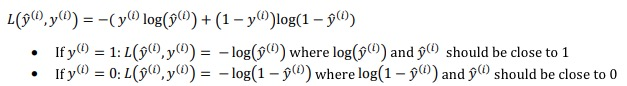分成y为0和1两种情况去理解这个函数，本质上就是对𝑦̂做对数处理而已。 因为对数处理后确实达到了Loss function的要求（我自己的理解）：1.值域是大于等于0的实数集。 2. 随着𝑦̂ 单调递减。y=𝑦̂ 的时候为0，反之趋向于∞。3. 是参数的凸函数（convex）4. 是y和𝑦̂的函数

3. Loss function是针对单个training example的，而Cost function是Loss Function的在所有training example上的均值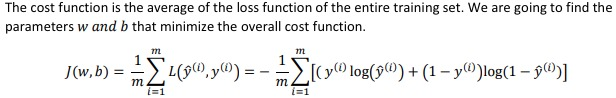在Machine learning里，没有引入Loss Function，其实有一个Loss Function，更好理解。

Gradient Descent的原理（Intuition）：按梯度最大的方向逼近最小值。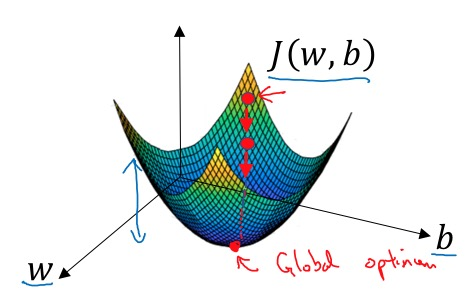1. Initialize $$w$$, $$b$$ to zero
2. repeat：

$$w\ :=w - w\frac{\partial J( w,b)}{\partial w}$$ $$b\ :=b - b\frac{\partial J( w,b)}{\partial b}$$

## Derivative with a Computation Graph

• 其实是就是复合函数的链式法则。
• 计算图左边的变量的偏导数依赖于右边的偏导数，右边的偏导数计算后，可以被左边的计算复用。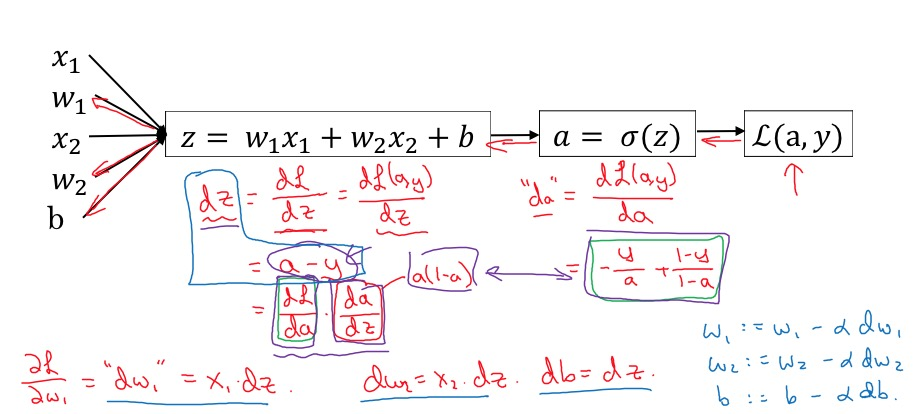## Gradient Descent on m Examples

Cost Function的偏导是Loss Function偏导的均值： $$\frac{\partial J(w,b)}{\partial w_j} =\frac{1}{m} \sum_{i=1}^{m} \frac{\partial \mathcal{L}(a^{(i)},y^{(i)})}{\partial w_j^{(i)}}$$

1. 求导过程 求导过程又通常是先forward propagation求cost function，然后再backward propagation求到w和b的倒数
2. 下降过程 使用到w和b的导数，迭代做梯度下降过程。

# Python and Vectorization

## Vectorization

1. 什么是Vectorization：将 for loop 尽可能转换为矩阵运算。举例： $$z = w^Tx + b$$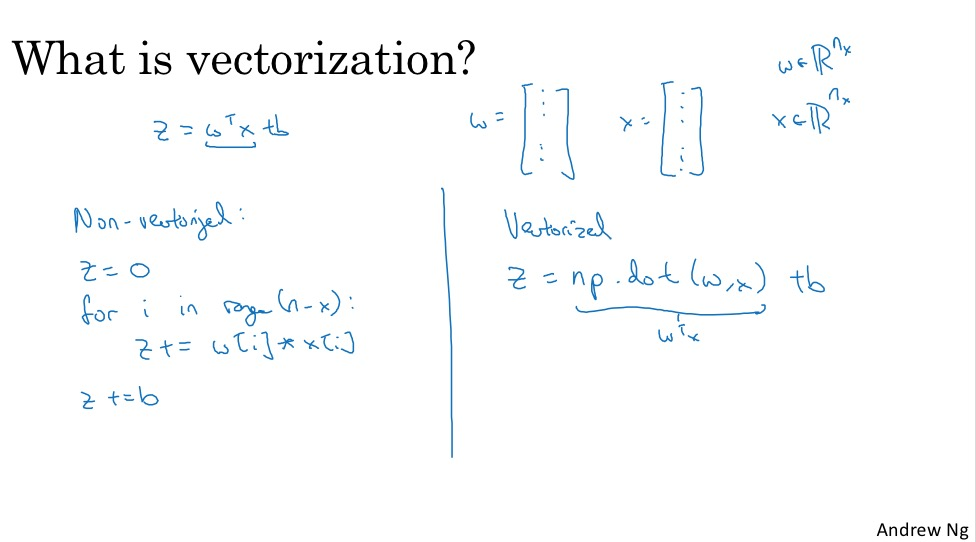2. vectorization的好处：conciser code, but faster execution 一个简单的对比实验：1,000,000大小的两个向量内积计算，for loop要比Vectorization快300倍。 在DeepLearning时代，vectorization是一项重要的技能。

3. SIMD Both CPU and GPU have parallelization instructions(i.e. SIMD, Signle Instruction Multiple Data)

## More vectorization Examples

1. 原则：whenever possible, avoid explict for-loops
2. 使用Element wised的矩阵运算，将函数作用在每个矩阵元素上，比如：
• np.exp()
• np.log()
• np.abs()
• np.maxium()
• 1/v
• v**2

## Vectorizing logistic Regression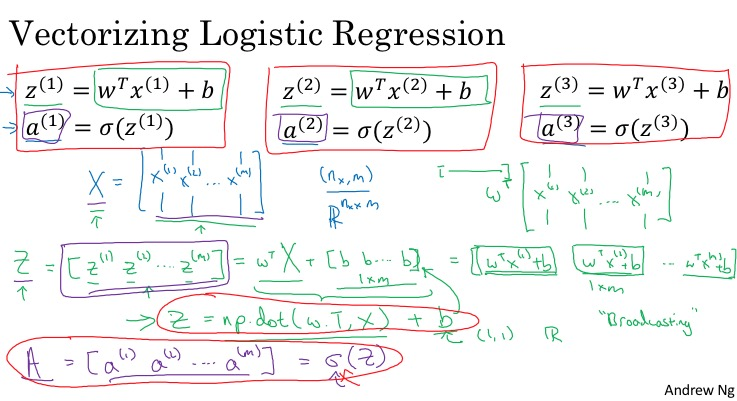$A = \sigma(w^T X + b) = (a^{(1)}, a^{(2)}, ..., a^{(m-1)}, a^{(m)})$

（我始终觉得A应该小写，毕竟还是一个行向量）

1. 首先，熟悉每个变量的记号和维度，必要的话，可以画出来，更直观。
2. 先从一个样本做向量化，再把m个样本的操作向量化。
3. for-loop里面是循环乘法，则向量化一定是一个乘法形式，若对于不确定乘法的左右关系，是否需转置，可以根据目标变量的维度推测。或者先乘起来，再根据目标变量看是否要转置。

## Vectorizing Logistic Regression’s Gradient Output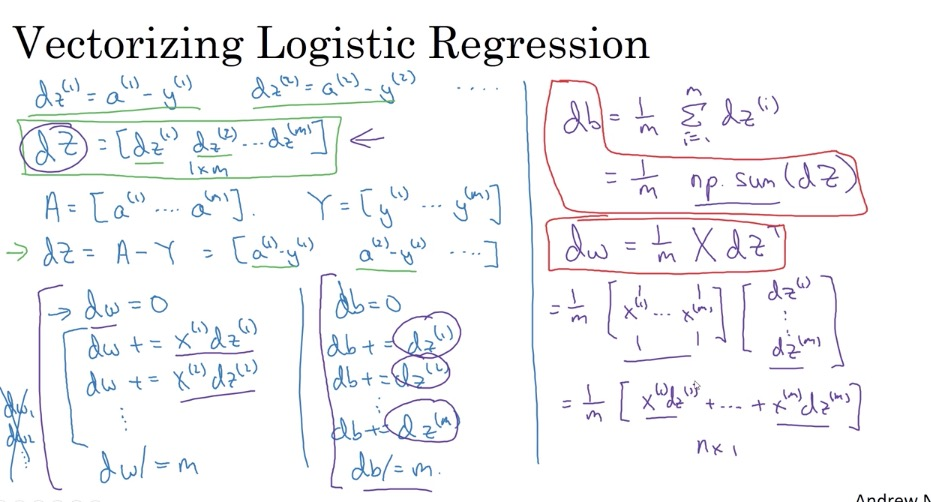$$\frac{\partial J}{\partial w} = \frac{1}{m}X(A-Y)^T$$ $$\frac{\partial J}{\partial b} = \frac{1}{m} \sum_{i=1}^m (a^{(i)}-y^{(i)})$$

1. 低纬度数组与高纬度共有的维度，元素个数必须一样，比如一个shape是(5,3)的数组可以和一个shape是(5,)的数组相加，但不能和一个(4,)的数组相加。

Andrew的一个经验：如果对某个数组的shape不确定，可以用reshape显式的调用一下，确保维度正确。

1. axis的数字，和数组的shape参数的索引是对应的。比如一个数组的shape是(5,6)，则代表5个row，6个column。即在shape中，row和column的个数的索引是0和1。也就第1个坐标，在shape中的第一个元素，索引是0，代表row的方向；第2个坐标，在shape中的第2个元素，索引是1，代表row的方向
2. 对于sum函数，axis指的是sum“沿着”的方向，经过计算，这个方向的维度因为求和后就消失了，比如sum(axis=0)代表是沿着“row”方向进行求和，
3. 当然axis可以是一个tupe，那就相当于沿着多个多个方向求和。
4. sum如果不传入axis参数，默认是对所有维度求和。

## A note on python/numpy vectors

rank 1 array问题：shape是(x,)的数组，既不是行向量，也不是列向量，没法参与正常的矩阵运算，应该总是使用(x,1)或(1,x)的shape来表示向量。但可以通过reshape方法将rank 1 array转换为行向量或列向量。（什么是rank，就是一个数组的维度）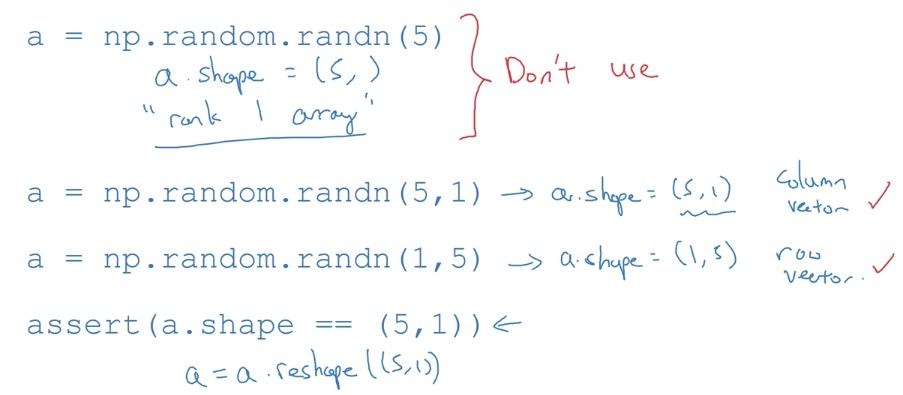## Explanation of logistic regression cost function (optional)

 Loss function其实就是对概率P(y x)取对数：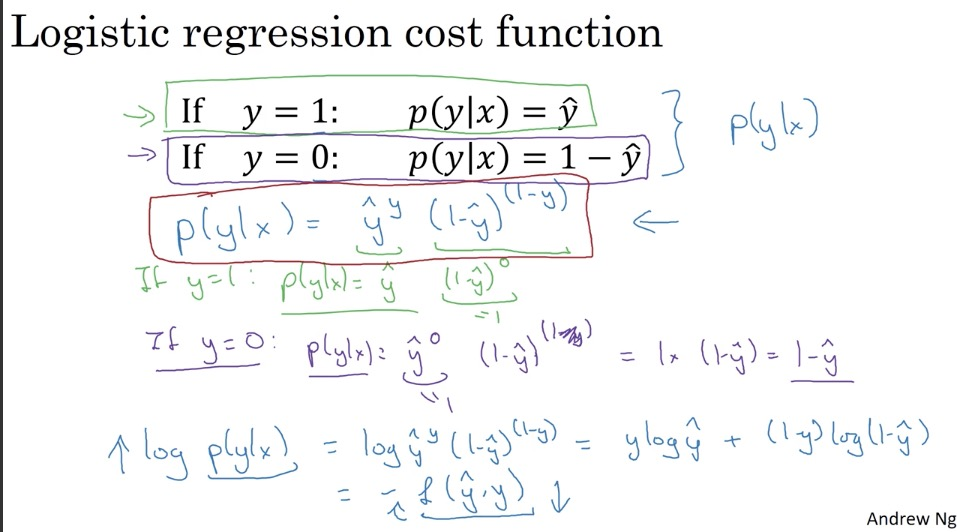Loss function越小，则取到和实际值y的概率越大。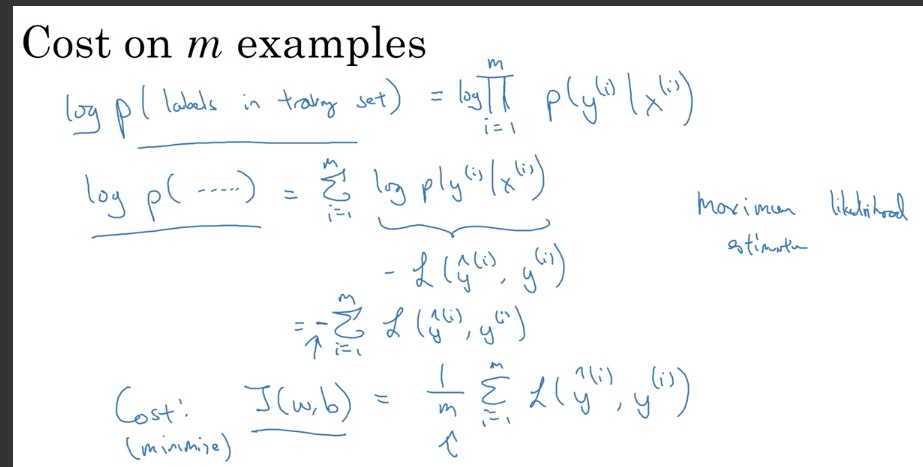# Heros of Deep Learning：Pieter Abbeel interview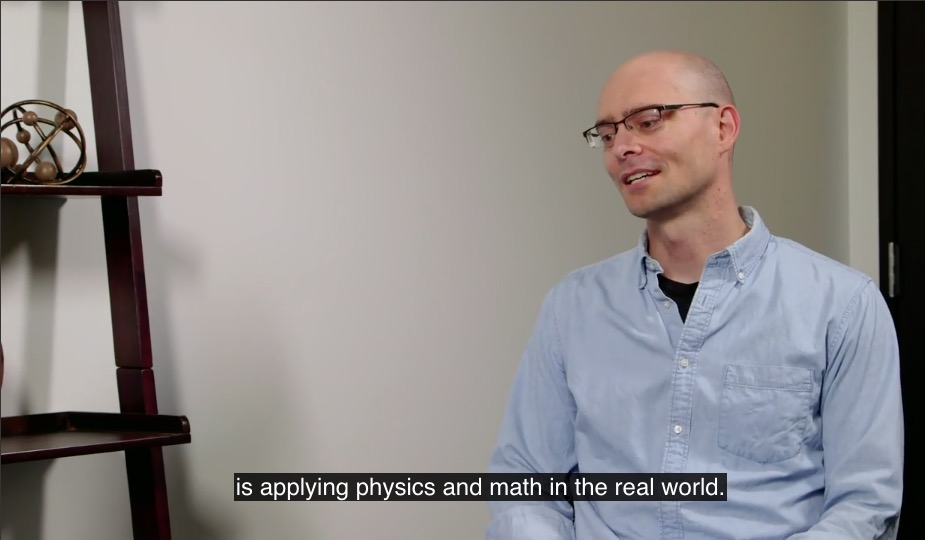1. Pieter Abbeel专注于deep reinforcement learning.

2. advice for people entrying AI
• A good time to enter AI. High demand.
• Not just read things or watch videos but try things out.
• With frameworks like TensorFlow, Chainer, Theano, PyTorch and so forth, it’s very easy to get going and get something up and running very quickly.
3. Andrew Ng: We live in good times. If people want to learn.

4. what are the things that deep reinforcement learning is already working really well at?
• learning to play games from pixels
• robot inventing walking, running, standing up with a single algorithm.

-------------------------